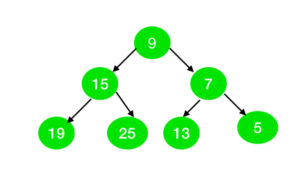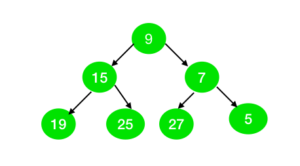# Check if all nodes of the Binary Tree can be represented as sum of two primes

• Last Updated : 19 Jul, 2022

Given a binary tree of N nodes with odd value. The task is to check whether all the nodes of the tree can be represented as the sum of the two prime numbers or not.

Examples:

Input:Output: Yes
Explanation:
All the nodes in the tree can be represented as the sum of two prime numbers as:
9 = 2 + 7
15 = 2 +13
7 = 2 + 5
19 = 2 + 17
25 = 2 + 23
13 = 11 + 2
5 = 2 + 3

Input:Output: No
Explanation:
The node with value 27 cannot be represented as the sum of two prime numbers.

Approach:

1. The idea is to use Goldbach’s Weak Conjecture which states that every odd number greater than 5 can be expressed as the sum of three primes.
2. To represent the odd number(say N) as a sum of two prime numbers, fix one prime number as 2 and if (N – 2) is also prime, then N can be represented as a sum of two prime numbers.
3. Check the above conditions for all the nodes in a tree. If any node doesn’t follow the above conditions, then print “No”, else print “Yes”.

## C++

 `// C++ program for the above approach` `#include ` `using` `namespace` `std;`   `// Function to create array to mark` `// whether element are prime or not` `void` `spf_array(``int` `arr[], ``int` `N)` `{` `    ``int` `i = 0;`   `    ``// Initially we set same value in` `    ``// array as a index of array.` `    ``for` `(i = 1; i <= N; i++) {` `        ``arr[i] = i;` `    ``}`   `    ``// Mark all even elements as 2` `    ``for` `(i = 2; i <= N; i = i + 2) {` `        ``arr[i] = 2;` `    ``}`   `    ``// Mark all the multiple of prime` `    ``// numbers as a non-prime` `    ``for` `(i = 3; i * i <= N; i++) {` `        ``if` `(arr[i] == i) {`   `            ``int` `j = 0;`   `            ``for` `(j = i * i; j <= N;` `                 ``j = j + i) {`   `                ``if` `(arr[j] == j) {` `                    ``arr[j] = i;` `                ``}` `            ``}` `        ``}` `    ``}` `}`   `// Tree Node` `struct` `node {` `    ``int` `val;` `    ``node* left;` `    ``node* right;` `};`   `// Function to create node of tree` `node* newnode(``int` `i)` `{` `    ``node* temp = NULL;` `    ``temp = ``new` `node();` `    ``temp->val = i;` `    ``temp->left = NULL;` `    ``temp->right = NULL;` `    ``return` `temp;` `}`   `// Function to check whether the` `// tree is prime or not` `int` `prime_tree(node* root, ``int` `arr[])` `{` `    ``int` `a = -1;` `    ``if` `(root != NULL) {`   `        ``// If element is not the sum of` `        ``// two prime then return 0` `        ``if` `(root->val <= 3` `            ``|| arr[root->val - 2]` `                   ``!= root->val - 2) {`   `            ``return` `0;` `        ``}` `    ``}`   `    ``if` `(root->left != NULL) {` `        ``a = prime_tree(root->left, arr);`   `        ``// If a is 0 then we don't need` `        ``// to check further` `        ``if` `(a == 0) {` `            ``return` `0;` `        ``}` `    ``}`   `    ``if` `(root->right != NULL) {`   `        ``a = prime_tree(root->right, arr);`   `        ``// If a is 0 then we don't need` `        ``// to check further` `        ``if` `(a == 0) {` `            ``return` `0;` `        ``}` `    ``}`   `    ``return` `1;` `}`   `// Driver Code` `int` `main()` `{`   `    ``// Given Tree` `    ``node* root = newnode(9);` `    ``root->right = newnode(7);` `    ``root->right->right = newnode(5);` `    ``root->right->left = newnode(13);` `    ``root->left = newnode(15);` `    ``root->left->left = newnode(19);` `    ``root->left->right = newnode(25);`   `    ``// Number of nodes in the tree` `    ``int` `n = 50;`   `    ``// Declare spf[] to store` `    ``// prime numbers` `    ``int` `brr[n + 1];` `    ``int` `i = 0;`   `    ``// Find prime numbers in spf[]` `    ``spf_array(brr, n + 1);`   `    ``// Function Call` `    ``if` `(prime_tree(root, brr)) {` `        ``cout << ``"Yes"` `<< endl;` `    ``}` `    ``else` `{` `        ``cout << ``"No"` `<< endl;` `    ``}` `}`

## Java

 `// Java program for the above approach` `import` `java.util.*;`   `class` `GFG{`   `// Function to create array to mark` `// whether element are prime or not` `static` `void` `spf_array(``int` `arr[], ``int` `N)` `{` `    ``int` `i = ``0``;`   `    ``// Initially we set same value in` `    ``// array as a index of array.` `    ``for``(i = ``1``; i <= N; i++)` `    ``{` `        ``arr[i] = i;` `    ``}`   `    ``// Mark all even elements as 2` `    ``for``(i = ``2``; i <= N; i = i + ``2``)` `    ``{` `        ``arr[i] = ``2``;` `    ``}`   `    ``// Mark all the multiple of prime` `    ``// numbers as a non-prime` `    ``for``(i = ``3``; i * i <= N; i++) ` `    ``{` `        ``if` `(arr[i] == i) ` `        ``{` `            ``int` `j = ``0``;` `            ``for``(j = i * i; j <= N; ` `                ``j = j + i)` `            ``{` `                ``if` `(arr[j] == j) ` `                ``{` `                    ``arr[j] = i;` `                ``}` `            ``}` `        ``}` `    ``}` `}`   `// Tree Node` `static` `class` `node` `{` `    ``int` `val;` `    ``node left;` `    ``node right;` `};`   `// Function to create node of tree` `static` `node newnode(``int` `i)` `{` `    ``node temp = ``null``;` `    ``temp = ``new` `node();` `    ``temp.val = i;` `    ``temp.left = ``null``;` `    ``temp.right = ``null``;` `    ``return` `temp;` `}`   `// Function to check whether ` `// the tree is prime or not` `static` `int` `prime_tree(node root, ``int` `arr[])` `{` `    ``int` `a = -``1``;` `    ``if` `(root != ``null``) ` `    ``{` `        `  `        ``// If element is not the sum ` `        ``// of two prime then return 0` `        ``if` `(root.val <= ``3` `|| ` `         ``arr[root.val - ``2``] != ` `             ``root.val - ``2``) ` `        ``{` `            ``return` `0``;` `        ``}` `    ``}` `    `  `    ``if` `(root.left != ``null``)` `    ``{` `        ``a = prime_tree(root.left, arr);`   `        ``// If a is 0 then we don't ` `        ``// need to check further` `        ``if` `(a == ``0``)` `        ``{` `            ``return` `0``;` `        ``}` `    ``}`   `    ``if` `(root.right != ``null``)` `    ``{` `        ``a = prime_tree(root.right, arr);`   `        ``// If a is 0 then we don't ` `        ``// need to check further` `        ``if` `(a == ``0``) ` `        ``{` `            ``return` `0``;` `        ``}` `    ``}` `    ``return` `1``;` `}`   `// Driver Code` `public` `static` `void` `main(String[] args)` `{` `    `  `    ``// Given Tree` `    ``node root = newnode(``9``);` `    ``root.right = newnode(``7``);` `    ``root.right.right = newnode(``5``);` `    ``root.right.left = newnode(``13``);` `    ``root.left = newnode(``15``);` `    ``root.left.left = newnode(``19``);` `    ``root.left.right = newnode(``25``);`   `    ``// Number of nodes in the tree` `    ``int` `n = ``50``;`   `    ``// Declare spf[] to store` `    ``// prime numbers` `    ``int` `[]brr = ``new` `int``[n + ``1``];` `    ``int` `i = ``0``;`   `    ``// Find prime numbers in spf[]` `    ``spf_array(brr, n);`   `    ``// Function Call` `    ``if` `(prime_tree(root, brr) == ``1``) ` `    ``{` `        ``System.out.print(``"Yes"` `+ ``"\n"``);` `    ``}` `    ``else` `    ``{` `        ``System.out.print(``"No"` `+ ``"\n"``);` `    ``}` `}` `}`   `// This code is contributed by Rohit_ranjan`

## Python3

 `# Python3 program for the above approach` `class` `Node:` `    `  `    ``def` `__init__(``self``, key):` `        `  `        ``self``.val ``=` `key` `        ``self``.left ``=` `None` `        ``self``.right ``=` `None`   `# Function to create array to mark` `# whether element are prime or not` `def` `spf_array(arr, N):` `    `  `    ``# Initially we set same value in` `    ``# array as a index of array.` `    ``for` `i ``in` `range``(``1``, N ``+` `1``):` `        ``arr[i] ``=` `i`   `    ``# Mark all even elements as 2` `    ``for` `i ``in` `range``(``2``, N ``+` `1``, ``2``):` `        ``arr[i] ``=` `2`   `    ``# Mark all the multiple of prime` `    ``# numbers as a non-prime` `    ``for` `i ``in` `range``(``3``, N ``+` `1``):` `        ``if` `i ``*` `i > N:` `            ``break` `        `  `        ``if` `(arr[i] ``=``=` `i):` `            ``for` `j ``in` `range``(``2` `*` `i, N, i):` `                ``if` `arr[j] ``=``=` `j:` `                    ``arr[j] ``=` `i`   `    ``return` `arr`   `# Function to check whether the` `# tree is prime or not` `def` `prime_tree(root, arr):` `    `  `    ``a ``=` `-``1` `    `  `    ``if` `(root !``=` `None``):` `        `  `        ``# If element is not the sum of` `        ``# two prime then return 0` `        ``if` `(root.val <``=` `3` `or`  `        ``arr[root.val ``-` `2``] !``=` `root.val ``-` `2``):` `            ``return` `0`   `    ``if` `(root.left !``=` `None``):` `        ``a ``=` `prime_tree(root.left, arr)`   `        ``# If a is 0 then we don't need` `        ``# to check further` `        ``if` `(a ``=``=` `0``):` `            ``return` `0`   `    ``if` `(root.right !``=` `None``):` `        ``a ``=` `prime_tree(root.right, arr)`   `        ``# If a is 0 then we don't need` `        ``# to check further` `        ``if` `(a ``=``=` `0``):` `            ``return` `0`   `    ``return` `1`   `# Driver Code` `if` `__name__ ``=``=` `'__main__'``:` `    `  `    ``# Given Tree` `    ``root ``=` `Node(``9``);` `    ``root.right ``=` `Node(``7``);` `    ``root.right.right ``=` `Node(``5``);` `    ``root.right.left ``=` `Node(``13``);` `    ``root.left ``=` `Node(``15``);` `    ``root.left.left ``=` `Node(``19``);` `    ``root.left.right ``=` `Node(``25``);`   `    ``# Number of nodes in the tree` `    ``n ``=` `50`   `    ``# Declare spf[] to store` `    ``# prime numbers` `    ``arr ``=` `[``0``] ``*` `(n ``+` `2``)`   `    ``# Find prime numbers in spf[]` `    ``brr ``=` `spf_array(arr, n ``+` `1``);`   `    ``# Function Call` `    ``if` `(prime_tree(root, brr)):` `        ``print``(``"Yes"``)` `    ``else``:` `        ``print``(``"No"``)`   `# This code is contributed by mohit kumar 29`

## C#

 `// C# program for the above approach` `using` `System;`   `class` `GFG{`   `// Function to create array to mark` `// whether element are prime or not` `static` `void` `spf_array(``int` `[]arr, ``int` `N)` `{` `    ``int` `i = 0;`   `    ``// Initially we set same value in` `    ``// array as a index of array.` `    ``for``(i = 1; i <= N; i++)` `    ``{` `        ``arr[i] = i;` `    ``}`   `    ``// Mark all even elements as 2` `    ``for``(i = 2; i <= N; i = i + 2)` `    ``{` `        ``arr[i] = 2;` `    ``}`   `    ``// Mark all the multiple of prime` `    ``// numbers as a non-prime` `    ``for``(i = 3; i * i <= N; i++) ` `    ``{` `        ``if` `(arr[i] == i) ` `        ``{` `            ``int` `j = 0;` `            ``for``(j = i * i; j <= N; ` `                ``j = j + i)` `            ``{` `                ``if` `(arr[j] == j) ` `                ``{` `                    ``arr[j] = i;` `                ``}` `            ``}` `        ``}` `    ``}` `}`   `// Tree Node` `class` `node` `{` `    ``public` `int` `val;` `    ``public` `node left;` `    ``public` `node right;` `};`   `// Function to create node of tree` `static` `node newnode(``int` `i)` `{` `    ``node temp = ``null``;` `    ``temp = ``new` `node();` `    ``temp.val = i;` `    ``temp.left = ``null``;` `    ``temp.right = ``null``;` `    ``return` `temp;` `}`   `// Function to check whether ` `// the tree is prime or not` `static` `int` `prime_tree(node root, ``int` `[]arr)` `{` `    ``int` `a = -1;` `    ``if` `(root != ``null``) ` `    ``{` `        `  `        ``// If element is not the sum ` `        ``// of two prime then return 0` `        ``if` `(root.val <= 3 || ` `        ``arr[root.val - 2] != ` `            ``root.val - 2) ` `        ``{` `            ``return` `0;` `        ``}` `    ``}` `    `  `    ``if` `(root.left != ``null``)` `    ``{` `        ``a = prime_tree(root.left, arr);`   `        ``// If a is 0 then we don't ` `        ``// need to check further` `        ``if` `(a == 0)` `        ``{` `            ``return` `0;` `        ``}` `    ``}`   `    ``if` `(root.right != ``null``)` `    ``{` `        ``a = prime_tree(root.right, arr);`   `        ``// If a is 0 then we don't ` `        ``// need to check further` `        ``if` `(a == 0) ` `        ``{` `            ``return` `0;` `        ``}` `    ``}` `    ``return` `1;` `}`   `// Driver Code` `public` `static` `void` `Main(String[] args)` `{` `    `  `    ``// Given Tree` `    ``node root = newnode(9);` `    ``root.right = newnode(7);` `    ``root.right.right = newnode(5);` `    ``root.right.left = newnode(13);` `    ``root.left = newnode(15);` `    ``root.left.left = newnode(19);` `    ``root.left.right = newnode(25);`   `    ``// Number of nodes in the tree` `    ``int` `n = 50;`   `    ``// Declare spf[] to store` `    ``// prime numbers` `    ``int` `[]brr = ``new` `int``[n + 1];`   `    ``// Find prime numbers in spf[]` `    ``spf_array(brr, n);`   `    ``// Function Call` `    ``if` `(prime_tree(root, brr) == 1) ` `    ``{` `        ``Console.Write(``"Yes"` `+ ``"\n"``);` `    ``}` `    ``else` `    ``{` `        ``Console.Write(``"No"` `+ ``"\n"``);` `    ``}` `}` `}`   `// This code is contributed by amal kumar choubey`

## Javascript

 ``

Output:

`Yes`

Time complexity : O(N * log(log N))
Auxiliary Space: O(N)

My Personal Notes arrow_drop_up
Recommended Articles
Page :